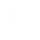Roman Numerals
Convert a Roman number to a numeric value and vice versa
Roman numerals table
 Roman     Numbers 0
Roman numerals table
Values:
I = 1
V = 5
X = 10
L = 50
C = 100
D = 500
M = 1000

Rules:
- The letters I, X, C can be repeated thrice in succession.
- V, L, D cannot be repeated or the number is considered to be invalid.
- If a lower value digit is written to the right of a higher value digit, it is added.
- If a lower value digit is written to the left of a higher value digit, it is subtracted.
- Only I, X, and C can be used as subtractive numerals.